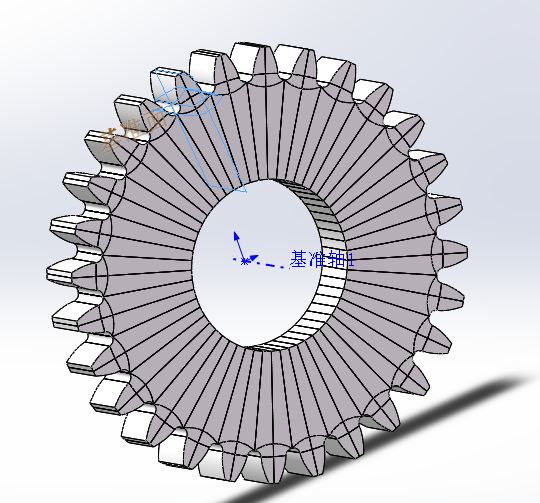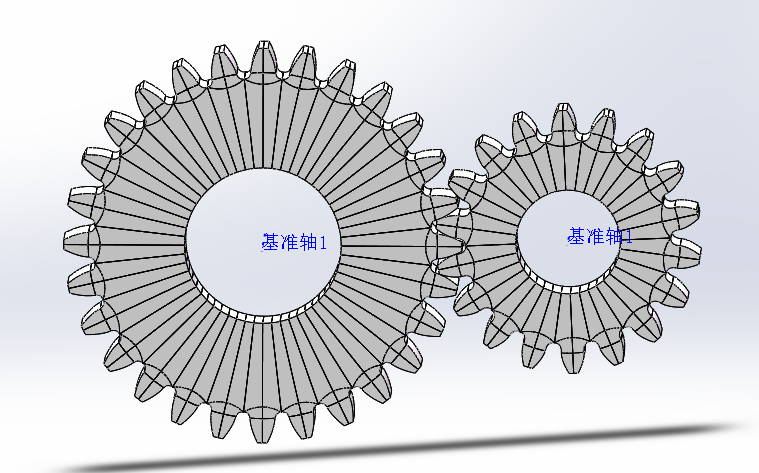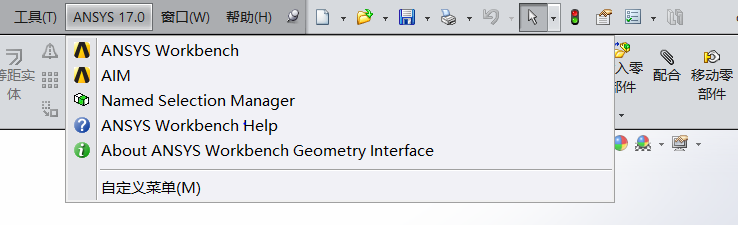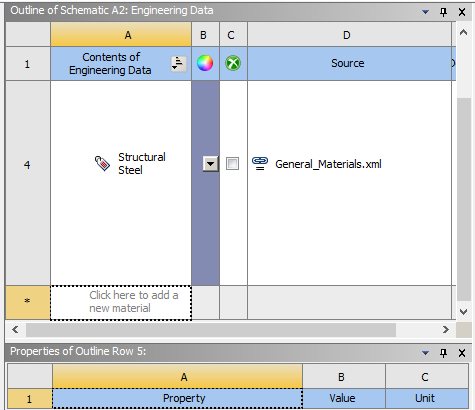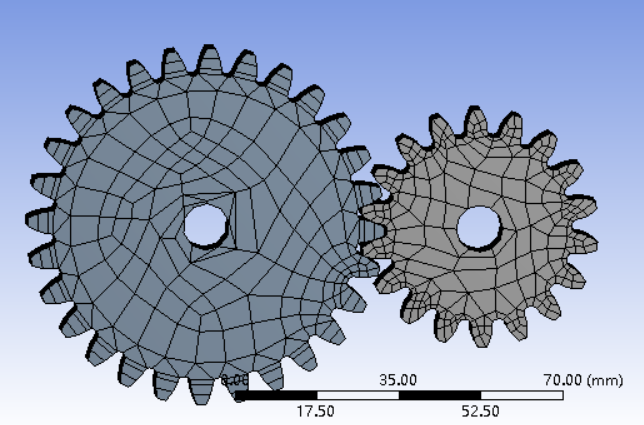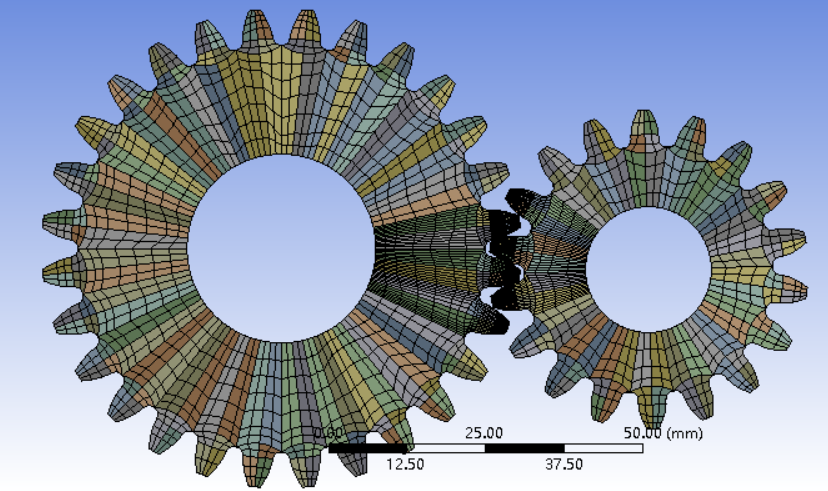# 齿轮组啮合过程仿真分析毕业论文

2020-04-08 02:04

Abstract

Gear is the most important part in the power transmission system. When the single and double gear teeth are engaged, the stiffness excitation caused by the elastic deformation of the gear will cause the gear to produce vibration and noise, so it is an important task of the gear dynamics to determine the meshing stiffness of the meshing tooth. In the range of gear stiffness (elasticity), mesh stiffness is proportional to the load and displacement of gear, that is, the force required for unit displacement. With the rapid development of science and technology, more and more computer technology applications and gear analysis, and finite element method is an approximate numerical method based on the rapid development of modern computer, which is used to solve the problem of partial differential equations with specific boundary conditions in mechanics and mathematics. The complicated stress distribution and deformation mechanism of the transmission gear are the main reasons for the difficulty in the gear design, while the finite element theory and the appearance of various finite element analysis software make the researchers do not need to do a lot of calculation and Research on the force of the gear, so that the force and deformation of the gear can be basically mastered, and the finite element can be used to make use of the finite element. The results of the meta computation are used to calculate the gear characteristics so as to better analyze the gears.

In this graduation design, the 3D modeling and Simulation of gear is built in SOLIDWORK based on the geometric parameters of the gear and the gear group is assembled. In order to make the finite element analysis of the model better, the model is segmented, and the model is introduced into the ANSYS Workbench to use the static dynamic module to carry out the finite element analysis of the three-dimensional model. Analysis, adding material, setting contact pair, dividing and refining grid, applying constraint, establishing finite element contact model, simulating gear transmission state, calculating gear meshing stiffness, checking calculation of gear meshing basic formula, calculating single and double meshing interval, changing its contact position through rotating gear, importing to workb The finite element analysis is carried out in ench, and the meshing stiffness of gears under different conditions is calculated, and the periodic change rules are analyzed.

Key words: 3D modeling; mesh generation; finite element analysis; meshing stiffness.

1.绪论 1

1.1课题背景及研究意义 1

1.2国内外的研究现状 1

1.3本文研究的工作 2

2.齿轮参数与刚度公式 2

2.1齿轮参数 2

2.2齿轮啮合刚度公式 3

3.直齿轮三维建模 4

3.1齿轮建模方案 4

3.2模型优化 5

3.3模型装配 6

3.4模型导入 7

4.齿轮啮合刚度计算 7

4.1导入材料与网格划分 8

4.2创建接触与施加约束 10

4.3仿真求解 11

4.4仿真结果验算 13

5.啮合刚度周期变化规律 14

5.1啮合位置的确定 14

5.2齿轮啮合刚度变化 16

5.3刚度变化规律 16

6.总结及后续工作 17

6.1论文总结 17

6.2存在的不足与后续工作 18

# 2.齿轮参数与刚度公式

## 2.1齿轮参数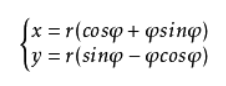（1）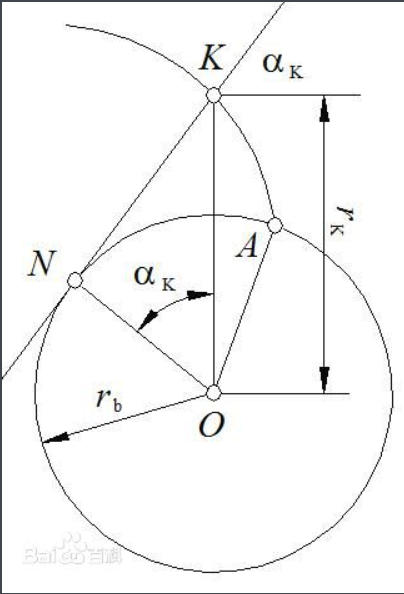## 2.2齿轮啮合刚度公式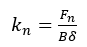（2）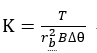（3）

# 3.直齿轮三维建模

 模数m/mm 压力角α/° 大齿轮齿数z1 小齿轮齿数z2 齿宽b/mm 2.54 20 28 18 10

## 3.1齿轮建模方案